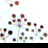# ArcGIS contruct a closed multipatch

3107
3
03-30-2016 02:47 PMby
New Contributor

Is there anybody know how to create a closed multipatch ? I create two cubes which I believe they are enclosed. but the ArcGIS 3D analyst told me it is not. I wondered if there is any criteria which should be used to create the closed cube . Please help !

the final result I need is to extract the overlapping part of the two cubes, In order to do this, the cubes have to be closed.3 RepliesOccasional Contributor II

A Multipatch is consideret to be closed if the Volume property of the Multipatch (from the IVolume interface) is not equal to zero. However, this property describes the enclosed volume of the Multipatch so it would be non-zero in case of highly deviated shapes too (twisted cubes, wrong direction/rotation of the triangles).

Here is a sample code:

Dim Points As PointClass() = New PointClass() _

{New PointClass() With {.X = 10.0#, .Y = 10.0#, .ZAware = True, .Z = 0.0#},

New PointClass() With {.X = 10.0#, .Y = 0.0#, .ZAware = True, .Z = 0.0#},

New PointClass() With {.X = 0.0#, .Y = 10.0#, .ZAware = True, .Z = 0.0#},

New PointClass() With {.X = 0.0#, .Y = 0.0#, .ZAware = True, .Z = 0.0#},

New PointClass() With {.X = 0.0#, .Y = 0.0#, .ZAware = True, .Z = 10.0#},

New PointClass() With {.X = 10.0#, .Y = 0.0#, .ZAware = True, .Z = 10.0#},

New PointClass() With {.X = 10.0#, .Y = 10.0#, .ZAware = True, .Z = 10.0#},

New PointClass() With {.X = 0.0#, .Y = 10.0#, .ZAware = True, .Z = 10.0#}}

Dim Triangles As New TriangleStripClass() With {.ZAware = True}

For Each N As Integer In New Integer() {0, 1, 2, 3, 4, 1, 5, 6, 4, 7, 2, 6, 0, 1}

Next

Dim Multipatch As New ESRI.ArcGIS.Geometry.MultiPatchClass() With {.ZAware = True}

Ákos HalmaibyEsri Contributor

Please go through the documentation below:

The Multipatch Geometry Type: An ESRI White Papter (December 2008)Occasional Contributor

A multipatch is not considered to be closed until the start point has been added at the end of the list of points.